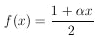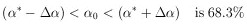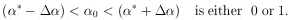### 2. INVERSE PROBABILITY

The more common problem facing a physicist is that he wishes to determine the final-state wave function from experimental measurements. For example, consider the decay of a spin-½ particle, the muon, which does not conserve parity. Because of angular-momentum conservation, we have the a priori knowledge thatHowever, the numerical value ofis some universal physical constant yet to be determined. We shall always use the subscript zero to denote the true physical value of the parameter under question. It is the job of the physicist to determine0. Usually the physicist does an experiment and quotes a result=* ±. The major portion of this report is devoted to the questions, What do we mean by* and? and What is the "best" way to calculate* and? These are questions of extreme importance to all physicists.

Crudely speaking,is the standard deviation,  and what the physicist usually means is that the "probability" of finding(the area under a Gaussian curve out to one standard deviation). The use of the word "probability" in the previous sentence would shock a mathematician. He would say the probability of havingThe kind of probability the physicist is talking about here we shall call inverse probability, in contrast to the direct probability used by the mathematician. Most physicists use the same word, probability, for the two completely different concepts: direct probability and inverse probability. In the remainder of this report we will conform to this sloppy physicist-usage of the word "probability."